ISEE Lower Level Quantitative : Converting units of measurement

Example Questions

1 2 4 Next →

Example Question #31 : Converting Units Of Measurement

How many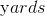are in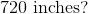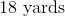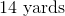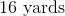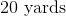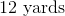Explanation:

To solve this problem we can make proportions.

We know that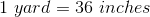, and we can useas our unknown.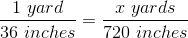Next, we want to cross multiply and divide to isolate theon one side.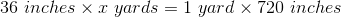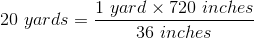The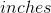will cancel and we are left with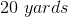Example Question #32 : Converting Units Of Measurement

How many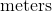are in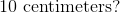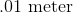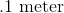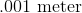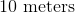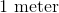Explanation:

To solve this problem we can make proportions.

We know that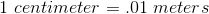and we can useas our unknown.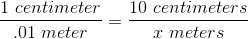Next, we want to cross multiply and divide to isolate the  on one side.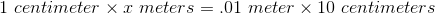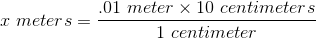The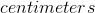will cancel and we are left with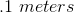Example Question #33 : Converting Units Of Measurement

How manyare in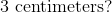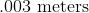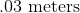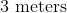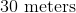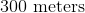Explanation:

To solve this problem we can make proportions.

We know thatand we can useas our unknown.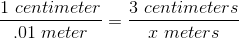Next, we want to cross multiply and divide to isolate the  on one side.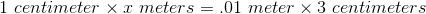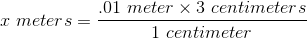Thewill cancel and we are left with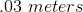Example Question #34 : Converting Units Of Measurement

How manyare in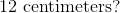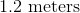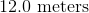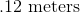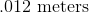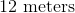Explanation:

To solve this problem we can make proportions.

We know thatand we can useas our unknown.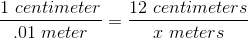Next, we want to cross multiply and divide to isolate the  on one side.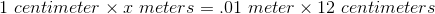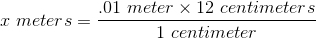Thewill cancel and we are left with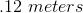Example Question #1 : Solving Word Problems With Multiple Unit Conversions

How many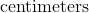are in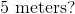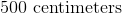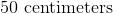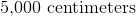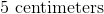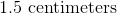Explanation:

To solve this problem we can make proportions.

We know thatand we can useas our unknown.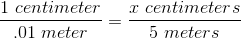Next, we want to cross multiply and divide to isolate the  on one side.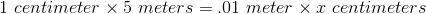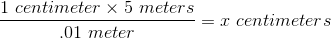The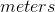will cancel and we are left with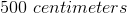Example Question #2 : Solving Word Problems With Multiple Unit Conversions

How many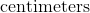are in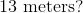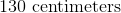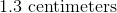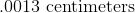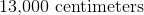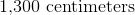Explanation:

To solve this problem we can make proportions.

We know thatand we can useas our unknown.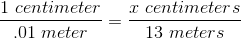Next, we want to cross multiply and divide to isolate the  on one side.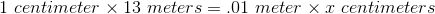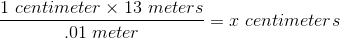Thewill cancel and we are left with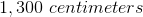1 2 4 Next →